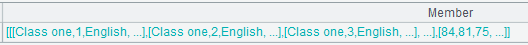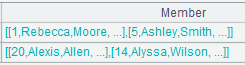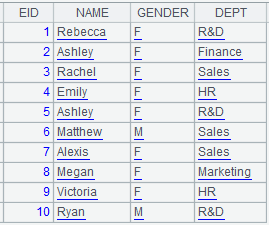# delete()

## T.delete()

 T.delete(k) 删除第k条记录 T.delete(p) 删除序号在p中的记录 T.delete(A) 删除在序列A中的记录

 T 序表 k 正整数，表示要删除的记录在序表中的位置 p n数列，定义需要删除记录的位置 A 序列，指定要删除的记录

 @n 返回删除的记录或排列

 A 1 =demo.query("select * from EMPLOYEE") 2 =A1.delete(1) 删除第一条记录 3 =A1.delete([2,4,6]) 删除第二、四、六条记录 4 =A1.select(EID>5) 5 =A1.delete(A4) 删除EID >5的记录 6 =A1.delete@n(1) 返回删除的记录

## A.delete()

 A.delete(k) 删除第k个成员 A.delete(p) 删除序号在p中的成员，p成员小于0时从后开始数

 A 序列 k 正整数，表示要删除的成员在序列中的位置 p n数列，定义需要删除的成员的位置

 @n 返回删除的记录或排列

 A 1 =["a","c","d","e","f"] 2 =A1.delete([2,4,5]) [a,d] 3 =demo.query("select * from STUDENTS") 4 =A3.delete@n(2)返回删除的排列 5 =["a","c","d","e","f"].delete([3,-5]) 返回结果：[c,e,f]，参数中的成员小于0时，从后往前数

## T.delete(P)

T.delete(P)

 T 实表 P 和T同构的排列

 @n 返回删除的记录

 A 1 =file("D:\\emp3.ctx") 2 =A1.create(#EID,NAME;EID) 创建以EID为排号键、分段键的组表基表 3 =demo.cursor("select EID,NAME from employee where EID< 10") 4 =A2.append(A3) 在基表中追加数据 5 =A2.attach(table3,GENDER) 给基表添加附表 6 =demo.cursor("select EID,GENDER from employee where EID< 10") 7 =A5.append(A6) 在附表中追加游标记录 8 =demo.query("select EID,GENDER from employee where EID =3 ") 返回EID为3的序表记录 9 =A5.delete(A8) 删除实表table3中EID为3的记录 10 =demo.query("select EID,NAME from employee where EID =2") 返回EID为2的序表记录 11 =A2.delete@n(A10)返回删除的记录

## T.delete(P)

T.delete(P)

 T 内表 P 和T同构的排列

 @n 返回删除掉的记录

 A 1 =demo. cursor ("select EID,NAME,GENDER from employee where EID< 10") 返回游标 2 =A1.memory() 返回内表3 =A2.keys(EID) 4 =demo.query("select EID,NAME,GENDER from employee where EID =3 ") 返回EID为3的序表记录 5 =A2.delete(A4) 删除EID为3的记录6 =demo.query("select EID,NAME,GENDER from employee where EID =5 ") 返回EID为5的序表记录 7 =A2.delete@n(A6) 返回删除的记录## T.delete(P)

T.delete(P)

 T 集群表 P 和T同构的排列

 A 1 =file("emp10.ctx":,["192.168.0.118:8281"]) 2 =A1.open() 打开集群组表 3 =demo.query("select EID,GENDER from employee where EID =3 ") 返回EID为3的序表记录 4 =A2.delete(A3) 删除实表table3中EID为3的记录 5 =A2.cursor().fetch()